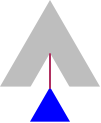# Functional Updaters

Imagine you have an n-ary tree which you wish to modify. For instance, the tree might represent the HTML of a Web page which you intend to change dynamically.

The simplest way to update the tree would be to copy it, incorporating the change while copying. Copying, however, takes time and space proportional to the size of the tree. If we wish to make several edits that are within close proximity of each other, perhaps we can devise a representation with lower complexity. Furthermore, we might wish to make these edits atomically, i.e., so the client sees only the result of all the edits and not the intermediate stages.

We can therefore consider representing trees in terms of a cursor, which reflects the locus around which we wish to modify the tree:A cursor represents an edge (shown in red) and has two parts: a representation of the (possibly empty) tree below that edge (shown in blue), and the (possibly empty) rest of the tree around that edge (shown in grey). The simplest representation of the part below is the subtree itself, but we have some choices in how we represent the rest of the tree, depending on which operations we wish to support.

We will represent a tree as follows:

```(define Tree\$ (or: Node\$ MtNode\$))
(define-struct: Node ([value : Any\$] [kids : (Listof: Tree\$)]))
(define-struct: MtNode ()) ;; read “Mt” as “empty”
```

We wish to support the following operations:

```find : (Tree\$ (Any\$ -> Boolean) -> Cursor\$)
```

This constructs a cursor by finding a point in a tree. The cursor represents the edge above the first node with a value for which the predicate produces `true`. We will assume that the search proceeds depth-first (so the cursor represents the edge above the leftmost node matching the predicate, and if there are multiple such nodes, it represents the edge above the topmost one).

Given a cursor, we can update the sub-tree at the bottom of the edge that it represents in constant time:

```update : (Cursor\$ (Tree\$ -> Tree\$) -> Cursor\$)
```

Note that because we have an explicit representation for an empty node, this operation can be used to insert, change, or delete a subtree. You would be wise to cover these operations in your test cases.

Once we are done performing updates, we can convert a cursor back into a tree:

```->tree : (Cursor\$ -> Tree\$)
```

The resulting tree should include only `Node\$`s, no `MtNode\$`s. However, if the resulting tree has no `Node\$`s, `->tree` should produce an `MtNode\$`.

In addition, we can navigate around a cursor:

```left : (Cursor\$ -> Cursor\$)
right : (Cursor\$ -> Cursor\$)
up : (Cursor\$ -> Cursor\$)
down : (Cursor\$ Integer\$ -> Cursor\$)
```

The `down` operation takes a numeric argument to indicate which child to select (with the index starting at `0`). Note that any of these operations might fail dynamically if the cursor is at the appropriate boundary. For instance, `left` and `right` should only be able to move between edges that share a common parent.

Critically, we want to make these four “motion” operations efficient—constant time or as close to it as possible. Can you design a data structure that achieves this? What is the complexity of the above operations that result from your representation choice?

## Part 2

Give a written explanation of how you designed your `Cursor` struct. Be sure to explain why you made the choices you made and how this affected the implementation of the various operations. Then do a big-O analysis of the complexity of these operations.

Note: It is important to make sure your analysis correctly describes your implementation. You will receive full credit on this section only for an accurate analysis of your code, even if your code is suboptimal.

## Extra Credit

There's no reason to stop with only one cursor: we can generalize to cursor sets. For instance, `find` can return the set of all cursors that match the given criterion (e.g., all the links on a Web page). In addition, sets of cursors can be combined with the usual set-theoretic operations. Extend your implementation to handle sets of cursors, being sure to consider and handle the subtleties that arise.

## What to turn in:

• A Racket file, `updater.rkt`, containing Racket code with your implementation of updater.

• A file, `analysis.[txt,pdf,doc`] with your answers for Part 2.
As always, these files must be in plain text, pdf, or, if you really must, Word format. If you use Word, we will accept only Word 2003 (`.doc`) files. If you are using Word 2007, you should export to a pdf. We will not accept Word 2007 (`.docx`) files because we cannot read them.